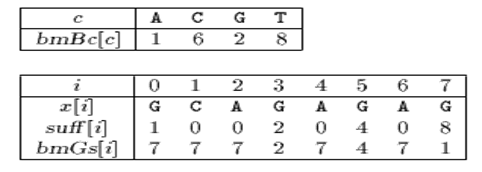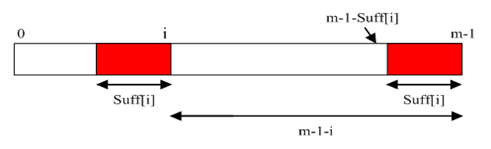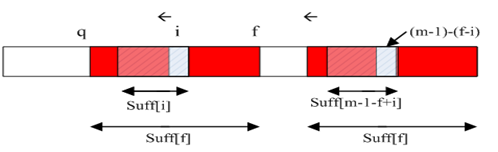# Boyer-Moore算法学习

#### 1、概述

BM算法在移动模式串的时候是从左到右，而进行比较的时候是从右到左的。

#### 2、BM算法思想

BM算法实际上包含两个并行的算法，坏字符算法和好后缀算法。这两种算法的目的就是让模式串每次向右移动尽可能大的距离（j+=x，x尽可能的大）。

###### 好后缀算法

Case1：模式串中有子串和好后缀安全匹配，则将最靠右的那个子串移动到好后缀的位置。继续进行匹配。Case2：如果不存在和好后缀完全匹配的子串，则在好后缀中找到具有如下特征的最长子串,使得P[m-s…m]=P[0…s]。说不清楚的看图。###### 坏字符算法

Case2：模式串中不存在坏字符。见图。###### 移动规则

BM算法的移动规则是：

BM算法是每次向右移动模式串的距离是，按照好后缀算法和坏字符算法计算得到的最大值。

shift（好后缀）和shift（坏字符）通过模式串的预处理数组的简单计算得到。好后缀算法的预处理数组是bmGs[]，坏字符算法的预处理数组是BmBc[]。

##### 3、代码分析
###### 定义

BM算法子串比较失配时，按坏字符算法计算模式串需要向右移动的距离，要借助BmBc数组。

BmBc数组的定义，分两种情况。

1、 字符在模式串中有出现。如下图，BmBc[‘k’]表示字符k在模式串中最后一次出现的位置，距离模式串串尾的长度。

2、 字符在模式串中没有出现：，如模式串中没有字符p，则BmBc[‘p’] = strlen(模式串)。BM算法子串比较失配时，按好后缀算法计算模式串需要向右移动的距离，要借助BmGs数组。

BmGs数组的下标是数字，表示字符在模式串中位置。

BmGs数组的定义，分三种情况。

1、 对应好后缀算法case1：如下图：i是好后缀之前的那个位置。2、 对应好后缀算法case2：如下图所示：3、 当都不匹配时，BmGs[i] = strlen（模式串）Suff数组的定义：suff[i] = 以i为边界, 与模式串后缀匹配的最大长度，即P[i-s...i]=P[m-s…m]如下图：###### 分析

1） BmGs[0…m-1] = m；（第三种情况）

2） 计算第二种情况下的BmGs[]值：

for（i=0；i

if（-1==i || Suff[i] == i+1）

for（；j < m-1-i；++j）

if（suff[j] == m）

BmGs[j] = m-1-i；

3） 计算第三种情况下BmGs[]值，可以覆盖前两种情况下的BmGs[]值：

for（i=0；i

BmGs[m-1-suff[i]] = m-1-i；Suff[]数组的计算方法。

Suff[m-1]=m；

for（i=m-2；i>=0；--i）{

q=i；

while（q>=0&&P[q]==P[m-1-i+q]）

--q；

Suff[i]=i-q；

}

i是当前正准备计算的suff[]值得那个位置。

f是上一个成功进行匹配的起始位置（不是每个位置都能进行成功匹配的，  实际上能够进行成功匹配的位置并不多）。

q是上一次进行成功匹配的失配位置。#### void preBmBc(char *x, int m, int bmBc[]) { int i; for (i = 0; i < ASIZE; ++i) bmBc[i] = m; for (i = 0; i < m - 1; ++i) bmBc[x[i]] = m - i - 1; } void suffixes(char *x, int m, int *suff) { int f, g, i; f = 0； suff[m - 1] = m; g = m - 1; for (i = m - 2; i >= 0; --i) { if (i > g && suff[i + m - 1 - f] < i - g) suff[i] = suff[i + m - 1 - f]; else { if (i < g) g = i; f = i; while (g >= 0 && x[g] == x[g + m - 1 - f]) --g; suff[i] = f - g; } } } void preBmGs(char *x, int m, int bmGs[]) { int i, j, suff[XSIZE]; suffixes(x, m, suff); for (i = 0; i < m; ++i) bmGs[i] = m; j = 0; for (i = m - 1; i >= 0; --i) if (suff[i] == i + 1) for (; j < m - 1 - i; ++j) if (bmGs[j] == m) bmGs[j] = m - 1 - i; for (i = 0; i <= m - 2; ++i) bmGs[m - 1 - suff[i]] = m - 1 - i; } void BM(char *x, int m, char *y, int n) { int i, j, bmGs[XSIZE], bmBc[ASIZE]; /* Preprocessing */ preBmGs(x, m, bmGs); preBmBc(x, m, bmBc); /* Searching */ j = 0; while (j <= n - m) { for (i = m - 1; i >= 0 && x[i] == y[i + j]; --i); if (i < 0) { OUTPUT(j); j += bmGs; } else j += MAX(bmGs[i], bmBc[y[i + j]] - m + 1 + i); } }

03-1410-112万+
09-211万+
06-221万+
09-195万+
10-201万+
08-151854
01-175906
05-193万+
04-07514
03-144558
05-223004
08-228013
04-171386
01-132845
12-055178
02-15188
©️2020 CSDN 皮肤主题: 大白 设计师:CSDN官方博客